Calculus II

Day10-Lecture 19

9 March 2017, Thursday 08:40

Previous Screenshot       Next Screenshot

The Second Derivative Test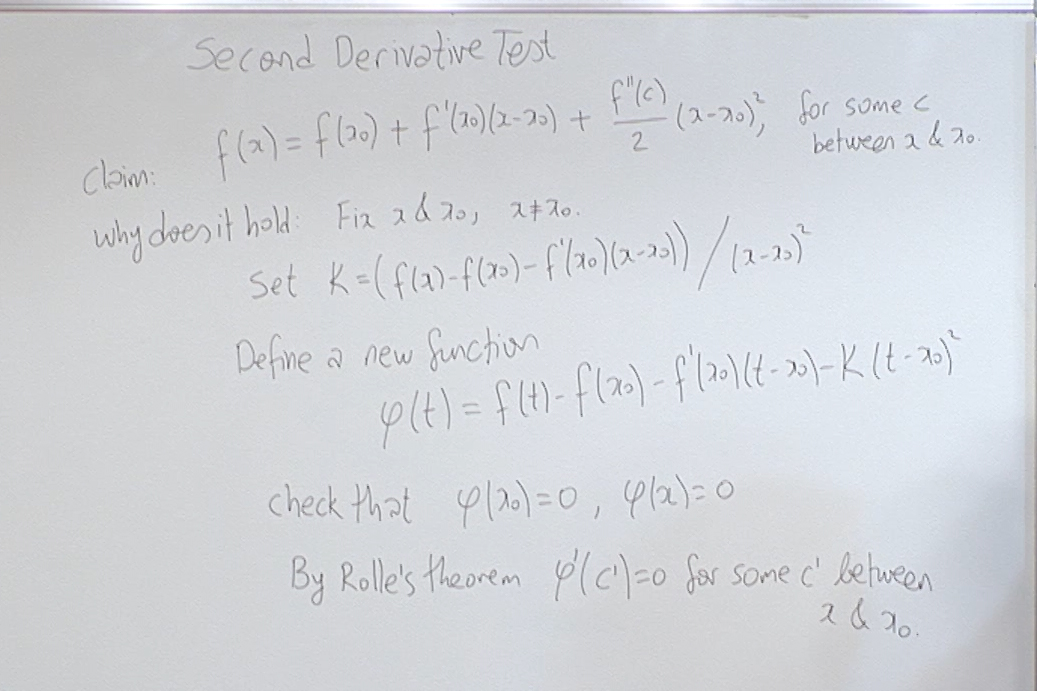We give the proof of Taylor's theorem as promised at the end of the last class.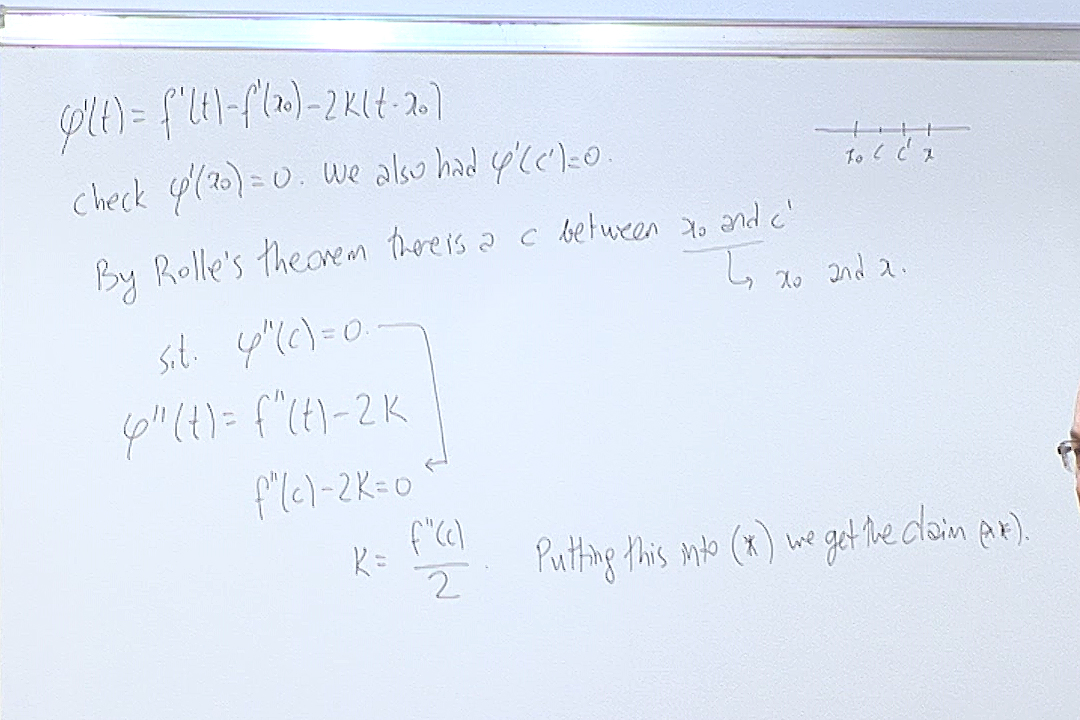This completes the proof.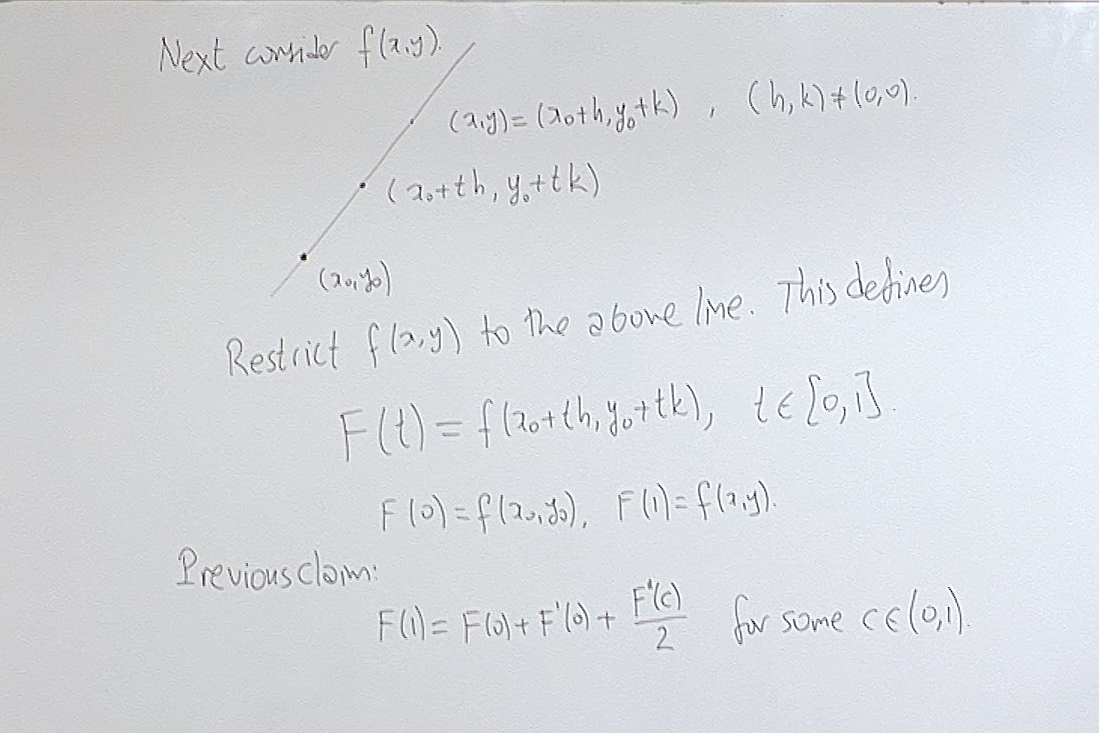We apply Taylor's theorem to $F(t)$.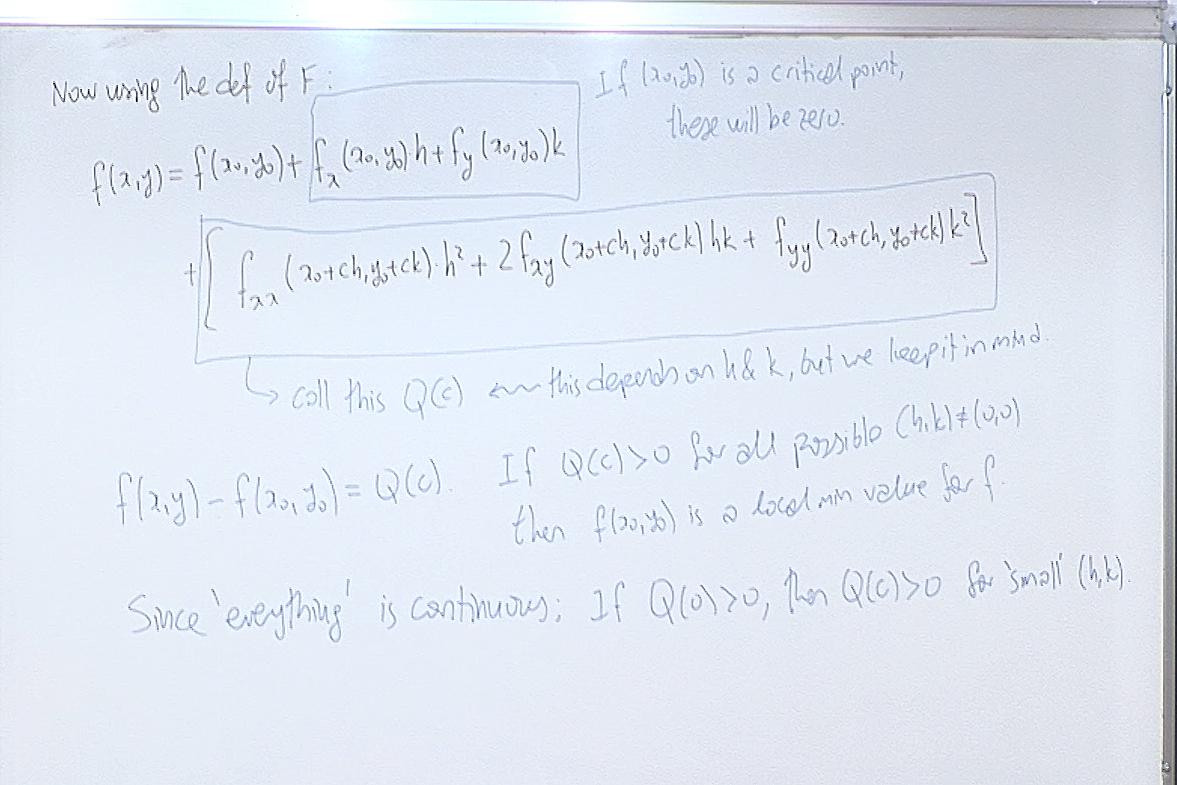We examine the implications on $f(x,y)$.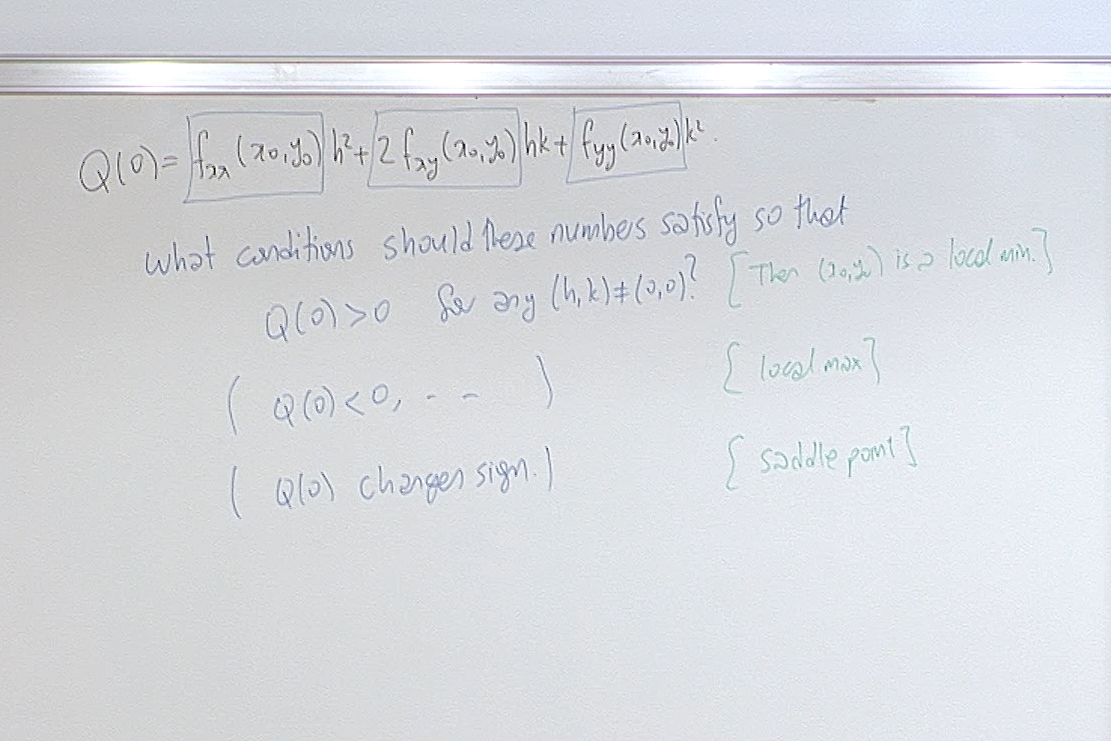We observe some consecuences pointing to min/max properties of $f(x,y)$.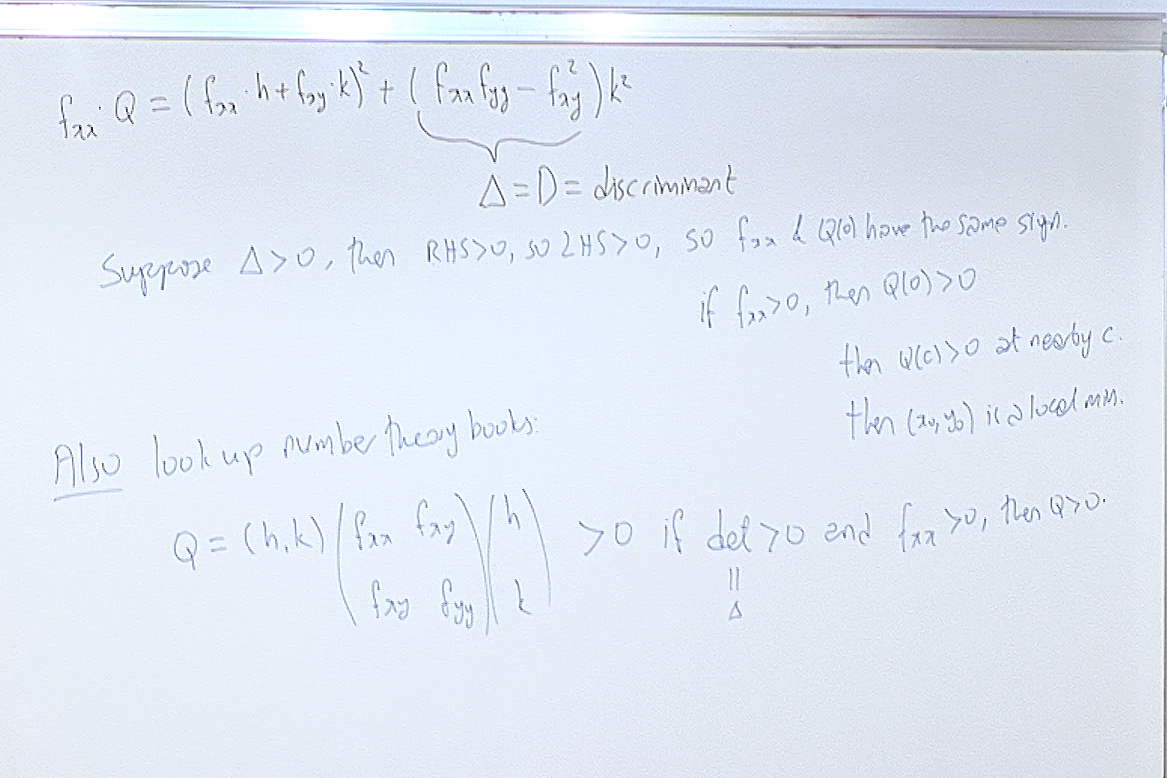If you are interested you can find a different presentation of these arguments in number theory books. The key term to search for is "binary forms".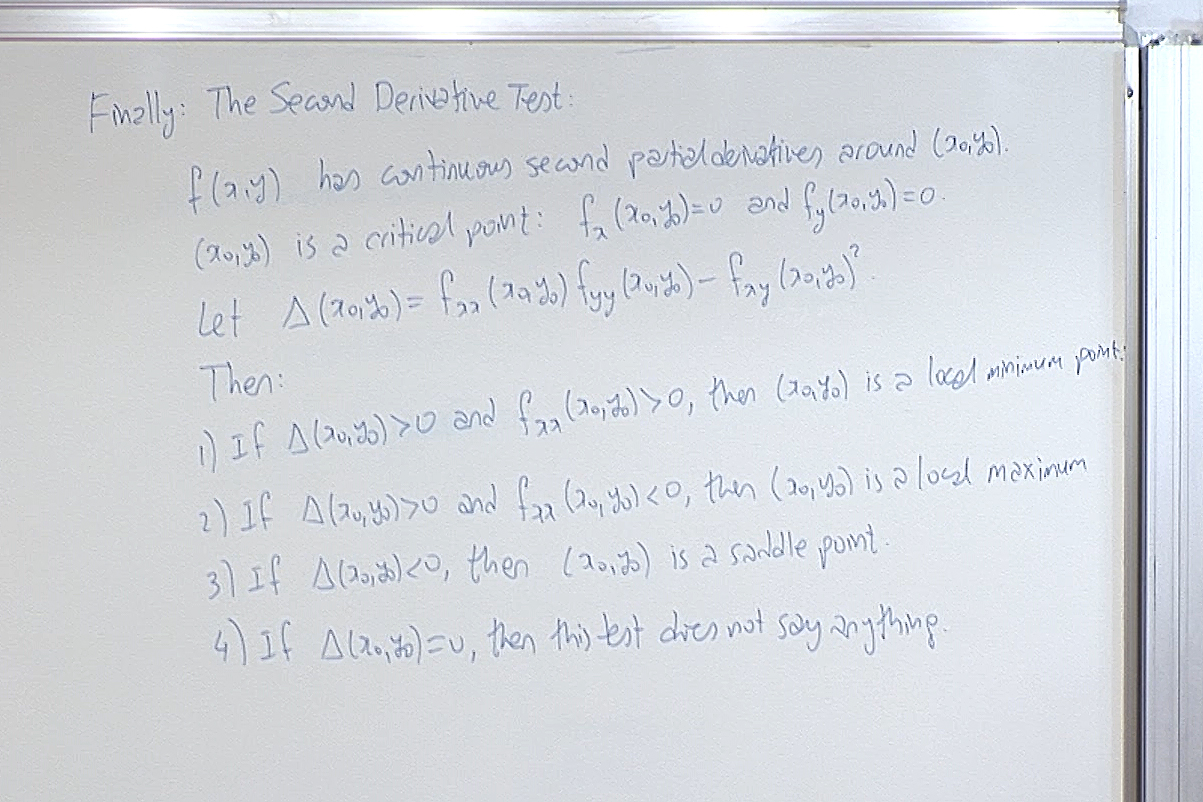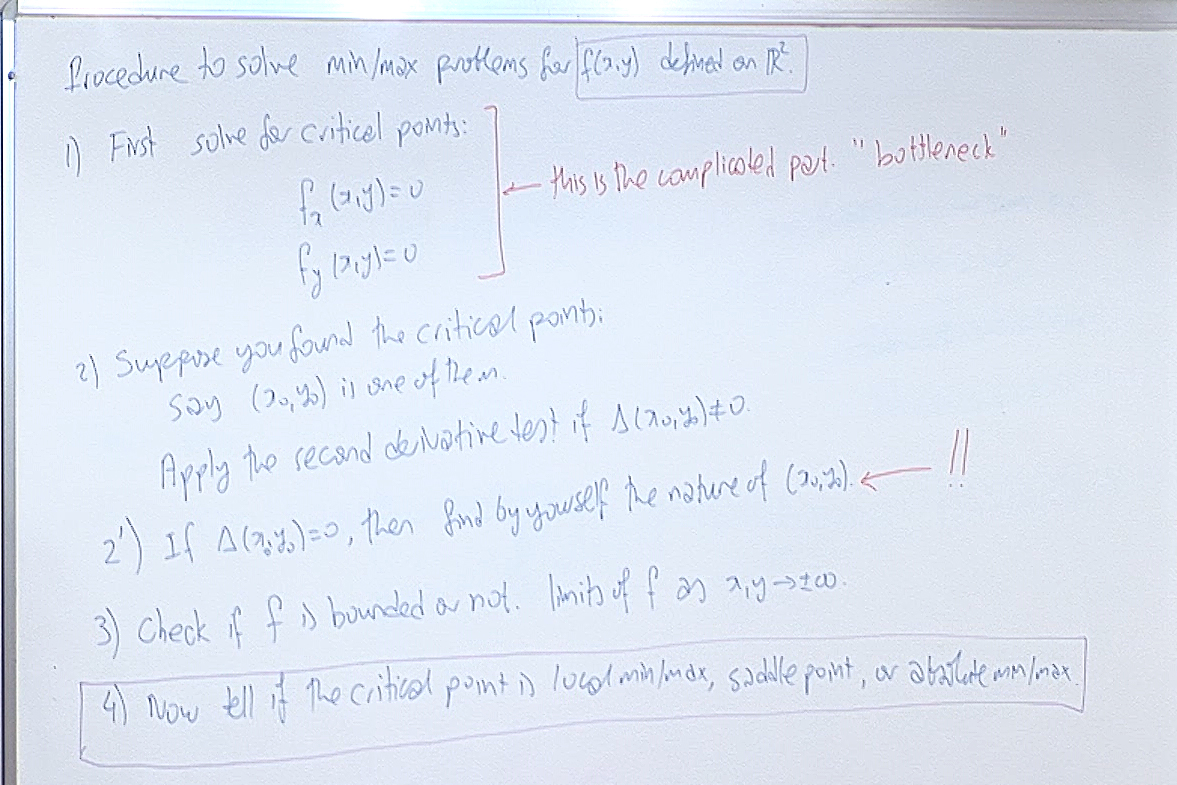For questions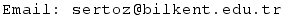Previous Screenshot       Next Screenshot